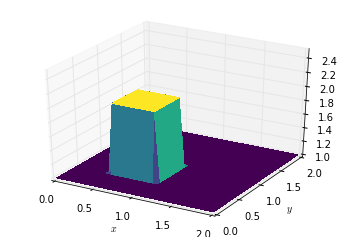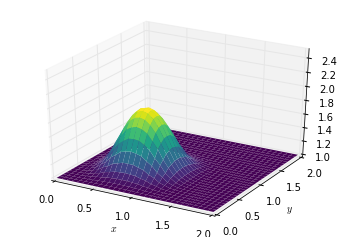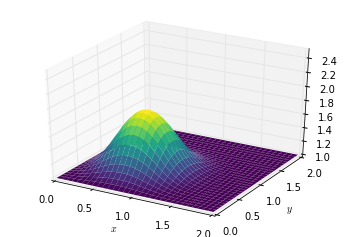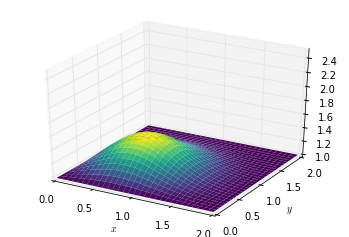Text provided under a Creative Commons Attribution license, CC-BY. All code is made available under the FSF-approved BSD-3 license. (c) Lorena A. Barba, Gilbert F. Forsyth 2017. Thanks to NSF for support via CAREER award #1149784.

# 12 steps to Navier–Stokes¶

You see where this is going ... we'll do 2D diffusion now and next we will combine steps 6 and 7 to solve Burgers' equation. So make sure your previous steps work well before continuing.

## Step 7: 2D Diffusion¶

And here is the 2D-diffusion equation:

$$\frac{\partial u}{\partial t} = \nu \frac{\partial ^2 u}{\partial x^2} + \nu \frac{\partial ^2 u}{\partial y^2}$$

You will recall that we came up with a method for discretizing second order derivatives in Step 3, when investigating 1-D diffusion. We are going to use the same scheme here, with our forward difference in time and two second-order derivatives.

$$\frac{u_{i,j}^{n+1} - u_{i,j}^n}{\Delta t} = \nu \frac{u_{i+1,j}^n - 2 u_{i,j}^n + u_{i-1,j}^n}{\Delta x^2} + \nu \frac{u_{i,j+1}^n-2 u_{i,j}^n + u_{i,j-1}^n}{\Delta y^2}$$

Once again, we reorganize the discretized equation and solve for $u_{i,j}^{n+1}$

$$\begin{split} u_{i,j}^{n+1} = u_{i,j}^n &+ \frac{\nu \Delta t}{\Delta x^2}(u_{i+1,j}^n - 2 u_{i,j}^n + u_{i-1,j}^n) \\ &+ \frac{\nu \Delta t}{\Delta y^2}(u_{i,j+1}^n-2 u_{i,j}^n + u_{i,j-1}^n) \end{split}$$
In :
import numpy
from matplotlib import pyplot, cm
from mpl_toolkits.mplot3d import Axes3D ##library for 3d projection plots
%matplotlib inline

In :
###variable declarations
nx = 31
ny = 31
nt = 17
nu = .05
dx = 2 / (nx - 1)
dy = 2 / (ny - 1)
sigma = .25
dt = sigma * dx * dy / nu

x = numpy.linspace(0, 2, nx)
y = numpy.linspace(0, 2, ny)

u = numpy.ones((ny, nx))  # create a 1xn vector of 1's
un = numpy.ones((ny, nx))

# set hat function I.C. : u(.5<=x<=1 && .5<=y<=1 ) is 2
u[int(.5 / dy):int(1 / dy + 1),int(.5 / dx):int(1 / dx + 1)] = 2

In :
fig = pyplot.figure()
ax = fig.gca(projection='3d')
X, Y = numpy.meshgrid(x, y)
surf = ax.plot_surface(X, Y, u, rstride=1, cstride=1, cmap=cm.viridis,
linewidth=0, antialiased=False)

ax.set_xlim(0, 2)
ax.set_ylim(0, 2)
ax.set_zlim(1, 2.5)

ax.set_xlabel('$x$')
ax.set_ylabel('$y$');$$\begin{split} u_{i,j}^{n+1} = u_{i,j}^n &+ \frac{\nu \Delta t}{\Delta x^2}(u_{i+1,j}^n - 2 u_{i,j}^n + u_{i-1,j}^n) \\ &+ \frac{\nu \Delta t}{\Delta y^2}(u_{i,j+1}^n-2 u_{i,j}^n + u_{i,j-1}^n) \end{split}$$
In :
###Run through nt timesteps
def diffuse(nt):
u[int(.5 / dy):int(1 / dy + 1),int(.5 / dx):int(1 / dx + 1)] = 2

for n in range(nt + 1):
un = u.copy()
u[1:-1, 1:-1] = (un[1:-1,1:-1] +
nu * dt / dx**2 *
(un[1:-1, 2:] - 2 * un[1:-1, 1:-1] + un[1:-1, 0:-2]) +
nu * dt / dy**2 *
(un[2:,1: -1] - 2 * un[1:-1, 1:-1] + un[0:-2, 1:-1]))
u[0, :] = 1
u[-1, :] = 1
u[:, 0] = 1
u[:, -1] = 1

fig = pyplot.figure()
ax = fig.gca(projection='3d')
surf = ax.plot_surface(X, Y, u[:], rstride=1, cstride=1, cmap=cm.viridis,
linewidth=0, antialiased=True)
ax.set_zlim(1, 2.5)
ax.set_xlabel('$x$')
ax.set_ylabel('$y$');


In :
diffuse(10)In :
diffuse(14)In :
diffuse(50)The video lesson that walks you through the details for Steps 5 to 8 is Video Lesson 6 on You Tube:

In :
from IPython.display import YouTubeVideo

from IPython.core.display import HTML